The Topological Classification of "closed surfaces."

Every connected closed and bounded surface is topologically equivalent to a sphere with handles and crosscaps attached.

Proof:
Step 1. Cut the surface into  "triangles."
Arrange these in the plane so that only edges that were adjacent on the surface are glued back together... staying in the plane. This gives a polygon in the plane when pairs of edges are  glued.
Thus... there are an even number of edges in this polygon.
Label the edges that are to be glued together as appropriate.

Step. 2. Eliminate A,-A: adjacent pairs with the same labels oriented in opposite directions.  [A toroidal pair]

This is a Java Applet created using GeoGebra from www.geogebra.org - it looks like you don't have Java installed, please go to www.java.com

Do this whenever possible after each subsequent step.

Step 3.Identify all the vertices:

Suppose P and Q are different vertices on the edge A. Then consider the next edge. If this edge is also A then either
(1)  the two edges are oriented in opposite directions- no, that can't be since we eliminated toroidal pairs, or

(2) they are oriented in the same direction- no that can't be because then the head and tail vertices of A would be the same... that is P=Q.
So ... The edge next to A is different from A, we'll call it B and assume A and B share the vertex P.

Now look for the other edge that matches B in the polygon. Draw an edge connecting the other vertex of B to Q. This forms a triangle with a new edge C. Cut of the triangle ABC and reattach it to the other B in the polygon. This makes one less P in the polygon and one more Q.

#### Step 4. Normalize Pairs A....A with the same orientation.

Cut from the head of A to the head of A along the edge C connecting the heads. Then paste the A's together, placing the A's inside the polygon and leaving adjacent C's with the same orientation.

As usual cancel toroidal pairs and repeat until all of these pairs have been normalized.

#### This is a Java Applet created using GeoGebra from www.geogebra.org - it looks like you don't have Java installed, please go to www.java.com

If after normalizing  the pairs that have the same orientation there are no other pairs, then the surface is a sphere with crosscaps attached, one for each pair of edges.
Step 5.  Normalize pairs  A.... A with opposite orientation.

Consider the edges between A and A.

If every edge between the heads of A  is paired with an edge also between the heads of A, then no vertex between the heads of A can be identified with a vertex between the tails of A.  BUT this can't be, since all the vertices are the same.

So... there must be at least one pair of edges R,  with opposite orrientations with one between the heads and the between the tails of A.

.....A.........R..........A(opp).........R(opp).....A....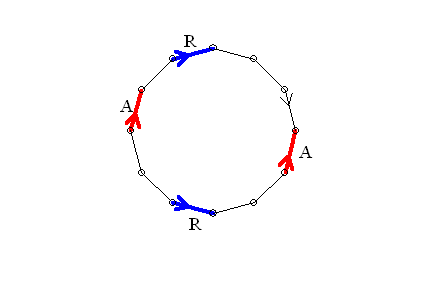Then cut from the head of C (opp) to the head of R with an edge S.
 Now cut between the heads of the R's with an edge C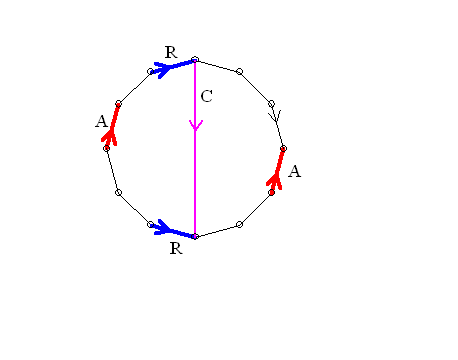and paste the A's together.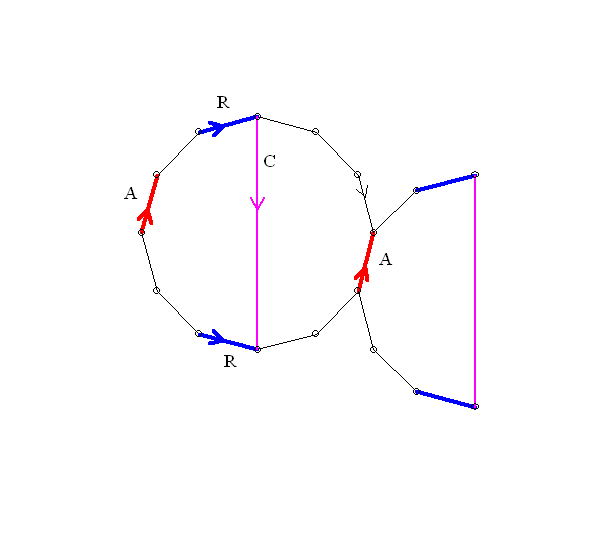This gives   .... RCR(opp).....C(opp)...   [The A's are now inside the polygon.]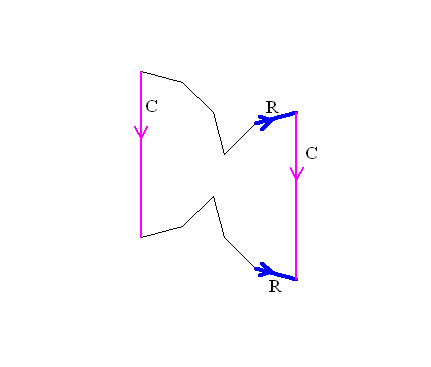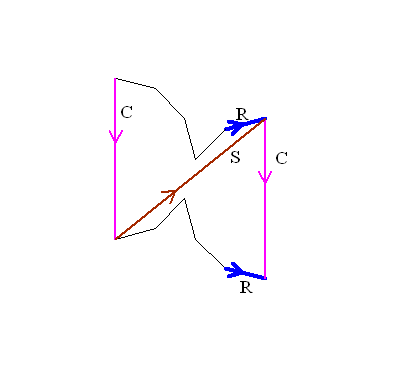Paste the two R edges together [so they are now interior]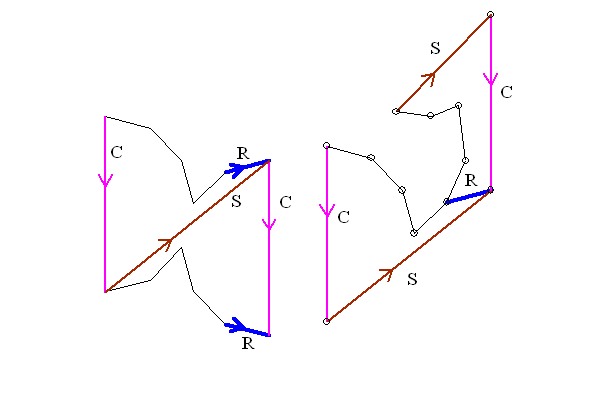and the edges are now SCS(opp)C(opp).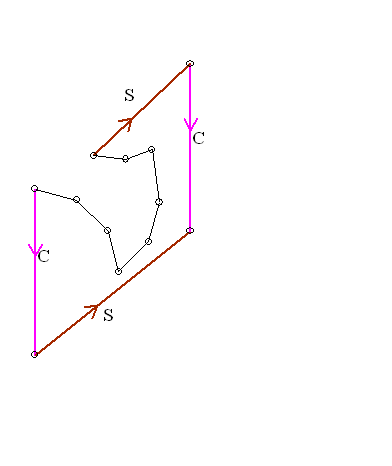In other words we now have found a handle!

Continue with this normalization until all edges have been normalized.

Conclusion: (1) If there were no step 4 pairs, then you have a sphere with as many handles to give the appropriate number of edges. [ n handles -> 2n edges.]
(2) If there were k - step 4 pairs and n  step 5 quadruples, then you have a sphere with k crosscaps and n handles and k +2n edges.

This classification determines the euler characteristic of each surface.
If the surface is orientable, it is a sphere with n handles, so V-E+R = 1 - 2n +1 = 2-2n.
For example, the torus has euler characteristic 2-2*1=0.
If the surface is non-orientable, then it
is a sphere with k crosscaps and n handles, so the euler characteristic is V-E+R = 1 - (k+2n) +1 =2 -2n -k.

Notice that a sphere with two cross caps has euler characteristic 0, the same as the torus. But this was the euler characteristic of the Klein Bottle. So we should be able to recognize the Klein bottle as a sphere with two cross caps.
This can be done by a single normalization of one pair of edges with the same  orientation.